xIntegerOverview

The integers are formed by the natural numbers (including 0) (0
0 (number)
0 is both a numberand the numerical digit used to represent that number in numerals.It fulfills a central role in mathematics as the additive identity of the integers, real numbers, and many other algebraic structures. As a digit, 0 is used as a placeholder in place value systems...

, 1, 2, 3, ...) together with the negatives of the non-zero natural numbers (−1, −2, −3, ...).They are known as Positive and Negative Integers respectively.Unanswered QuestionsHow do u - an intergerDiscussionsPowersCan every integer other than 0 be written as a power?The difference of any 2 negative numbers is negative or positiveEncyclopediaThe integers are formed by the natural numbers (including 0) (0
0 (number)
0 is both a numberand the numerical digit used to represent that number in numerals.It fulfills a central role in mathematics as the additive identity of the integers, real numbers, and many other algebraic structures. As a digit, 0 is used as a placeholder in place value systems...

, 1, 2, 3, ...) together with the negatives of the non-zero natural numbers (−1, −2, −3, ...).They are known as Positive and Negative Integers respectively. Viewed as a subset of the real number
Real number
In mathematics, a real number is a value that represents a quantity along a continuum, such as -5 , 4/3 , 8.6 , √2 and π...

s, they are numbers that can be written without a fractional or decimal component, and fall within the set {..., −2, −1, 0, 1, 2, ...}. For example, 21, 4, and −2048 are integers; 9.75, 5½, and 14% are not integers.

The set of all integers is often denoted by a boldface Z (or blackboard bold
Blackboard bold
Blackboard bold is a typeface style that is often used for certain symbols in mathematical texts, in which certain lines of the symbol are doubled. The symbols usually denote number sets...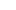, Unicode
Unicode
Unicode is a computing industry standard for the consistent encoding, representation and handling of text expressed in most of the world's writing systems...

U+2124 ), which stands for Zahlen (German
German language
German is a West Germanic language, related to and classified alongside English and Dutch. With an estimated 90 – 98 million native speakers, German is one of the world's major languages and is the most widely-spoken first language in the European Union....

for numbers, pronounced ˈtsaːlən). The set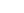is the finite set of integers modulo
Modular arithmetic
In mathematics, modular arithmetic is a system of arithmetic for integers, where numbers "wrap around" after they reach a certain value—the modulus....

n (for example,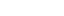).

The integers (with addition as operation) form the smallest group
Group (mathematics)
In mathematics, a group is an algebraic structure consisting of a set together with an operation that combines any two of its elements to form a third element. To qualify as a group, the set and the operation must satisfy a few conditions called group axioms, namely closure, associativity, identity...

containing the additive monoid of the natural number
Natural number
In mathematics, the natural numbers are the ordinary whole numbers used for counting and ordering . These purposes are related to the linguistic notions of cardinal and ordinal numbers, respectively...

s. Like the natural numbers, the integers form a countably infinite
Countable set
In mathematics, a countable set is a set with the same cardinality as some subset of the set of natural numbers. A set that is not countable is called uncountable. The term was originated by Georg Cantor...

set.

In algebraic number theory
Algebraic number theory
Algebraic number theory is a major branch of number theory which studies algebraic structures related to algebraic integers. This is generally accomplished by considering a ring of algebraic integers O in an algebraic number field K/Q, and studying their algebraic properties such as factorization,...

, these commonly understood integers, embedded in the field
Field (mathematics)
In abstract algebra, a field is a commutative ring whose nonzero elements form a group under multiplication. As such it is an algebraic structure with notions of addition, subtraction, multiplication, and division, satisfying certain axioms...

of rational number
Rational number
In mathematics, a rational number is any number that can be expressed as the quotient or fraction a/b of two integers, with the denominator b not equal to zero. Since b may be equal to 1, every integer is a rational number...

s, are referred to as rational integers to distinguish them from the more broadly defined algebraic integer
Algebraic integer
In number theory, an algebraic integer is a complex number that is a root of some monic polynomial with coefficients in . The set of all algebraic integers is closed under addition and multiplication and therefore is a subring of complex numbers denoted by A...

s (but with "rational" meaning "quotient of integers", this attempt at precision suffers from circularity).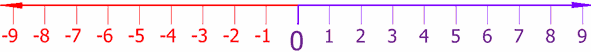## Algebraic properties

Like the natural numbers, Z is closed
Closure (mathematics)
In mathematics, a set is said to be closed under some operation if performance of that operation on members of the set always produces a unique member of the same set. For example, the real numbers are closed under subtraction, but the natural numbers are not: 3 and 8 are both natural numbers, but...

under the operations
Binary operation
In mathematics, a binary operation is a calculation involving two operands, in other words, an operation whose arity is two. Examples include the familiar arithmetic operations of addition, subtraction, multiplication and division....

of addition
Addition
Addition is a mathematical operation that represents combining collections of objects together into a larger collection. It is signified by the plus sign . For example, in the picture on the right, there are 3 + 2 apples—meaning three apples and two other apples—which is the same as five apples....

and multiplication
Multiplication
Multiplication is the mathematical operation of scaling one number by another. It is one of the four basic operations in elementary arithmetic ....

, that is, the sum and product of any two integers is an integer. However, with the inclusion of the negative natural numbers, and, importantly, zero
0 (number)
0 is both a numberand the numerical digit used to represent that number in numerals.It fulfills a central role in mathematics as the additive identity of the integers, real numbers, and many other algebraic structures. As a digit, 0 is used as a placeholder in place value systems...

, Z (unlike the natural numbers) is also closed under subtraction
Subtraction
In arithmetic, subtraction is one of the four basic binary operations; it is the inverse of addition, meaning that if we start with any number and add any number and then subtract the same number we added, we return to the number we started with...

. Z is not closed under division
Division (mathematics)
right|thumb|200px|20 \div 4=5In mathematics, especially in elementary arithmetic, division is an arithmetic operation.Specifically, if c times b equals a, written:c \times b = a\,...

, since the quotient of two integers (e.g., 1 divided by 2), need not be an integer. Although the natural numbers are closed under exponentiation
Exponentiation
Exponentiation is a mathematical operation, written as an, involving two numbers, the base a and the exponent n...

, the integers are not (since the result can be a fraction when the exponent is negative).

The following lists some of the basic properties of addition and multiplication for any integers a, b and c.
 Addition Multiplication ClosureClosure (mathematics)In mathematics, a set is said to be closed under some operation if performance of that operation on members of the set always produces a unique member of the same set. For example, the real numbers are closed under subtraction, but the natural numbers are not: 3 and 8 are both natural numbers, but...: a + b   is an integer a × b   is an integer AssociativityAssociativityIn mathematics, associativity is a property of some binary operations. It means that, within an expression containing two or more occurrences in a row of the same associative operator, the order in which the operations are performed does not matter as long as the sequence of the operands is not...: a + (b + c)  =  (a + b) + c a × (b × c)  =  (a × b) × c CommutativityCommutativityIn mathematics an operation is commutative if changing the order of the operands does not change the end result. It is a fundamental property of many binary operations, and many mathematical proofs depend on it...: a + b  =  b + a a × b  =  b × a Existence of an identity elementIdentity elementIn mathematics, an identity element is a special type of element of a set with respect to a binary operation on that set. It leaves other elements unchanged when combined with them...: a + 0  =  a a × 1  =  a Existence of inverse elementInverse elementIn abstract algebra, the idea of an inverse element generalises the concept of a negation, in relation to addition, and a reciprocal, in relation to multiplication. The intuition is of an element that can 'undo' the effect of combination with another given element...s: a + (−a)  =  0 An inverse element usually does not exist at all. DistributivityDistributivityIn mathematics, and in particular in abstract algebra, distributivity is a property of binary operations that generalizes the distributive law from elementary algebra.For example:...: and No zero divisorZero divisorIn abstract algebra, a nonzero element a of a ring is a left zero divisor if there exists a nonzero b such that ab = 0. Similarly, a nonzero element a of a ring is a right zero divisor if there exists a nonzero c such that ca = 0. An element that is both a left and a right zero divisor is simply...s: If a × b = 0, then a = 0 or b = 0 (or both)

In the language of abstract algebra
Abstract algebra
Abstract algebra is the subject area of mathematics that studies algebraic structures, such as groups, rings, fields, modules, vector spaces, and algebras...

, the first five properties listed above for addition say that Z under addition is an abelian group
Abelian group
In abstract algebra, an abelian group, also called a commutative group, is a group in which the result of applying the group operation to two group elements does not depend on their order . Abelian groups generalize the arithmetic of addition of integers...

. As a group under addition, Z is a cyclic group
Cyclic group
In group theory, a cyclic group is a group that can be generated by a single element, in the sense that the group has an element g such that, when written multiplicatively, every element of the group is a power of g .-Definition:A group G is called cyclic if there exists an element g...

, since every nonzero integer can be written as a finite sum 1 + 1 + ... + 1 or (−1) + (−1) + ... + (−1). In fact, Z under addition is the only infinite cyclic group, in the sense that any infinite cyclic group is isomorphic
Group isomorphism
In abstract algebra, a group isomorphism is a function between two groups that sets up a one-to-one correspondence between the elements of the groups in a way that respects the given group operations. If there exists an isomorphism between two groups, then the groups are called isomorphic...

to Z.

The first four properties listed above for multiplication say that Z under multiplication is a commutative monoid. However not every integer has a multiplicative inverse; e.g. there is no integer x such that because the left hand side is even, while the right hand side is odd. This means that Z under multiplication is not a group.

All the rules from the above property table, except for the last, taken together say that Z together with addition and multiplication is a commutative ring
Ring (mathematics)
In mathematics, a ring is an algebraic structure consisting of a set together with two binary operations usually called addition and multiplication, where the set is an abelian group under addition and a semigroup under multiplication such that multiplication distributes over addition...

with unity. Adding the last property says that Z is an integral domain. In fact, Z provides the motivation for defining such a structure.

The lack of multiplicative inverses, which is equivalent to the fact that Z is not closed under division, means that Z is not a field
Field (mathematics)
In abstract algebra, a field is a commutative ring whose nonzero elements form a group under multiplication. As such it is an algebraic structure with notions of addition, subtraction, multiplication, and division, satisfying certain axioms...

. The smallest field containing the integers is the field of rational number
Rational number
In mathematics, a rational number is any number that can be expressed as the quotient or fraction a/b of two integers, with the denominator b not equal to zero. Since b may be equal to 1, every integer is a rational number...

s. The process of constructing the rationals from the integers can be mimicked to form the field of fractions
Field of fractions
In abstract algebra, the field of fractions or field of quotients of an integral domain is the smallest field in which it can be embedded. The elements of the field of fractions of the integral domain R have the form a/b with a and b in R and b ≠ 0...

of any integral domain.

Although ordinary division is not defined on Z, it does possess an important property called the division algorithm
Division algorithm
In mathematics, and more particularly in arithmetic, the usual process of division of integers producing a quotient and a remainder can be specified precisely by a theorem stating that these exist uniquely with given properties. An integer division algorithm is any effective method for producing...

: that is, given two integers a and b with b ≠ 0, there exist unique integers q and r such that and 0 ≤ r < | b |, where | b | denotes the absolute value
Absolute value
In mathematics, the absolute value |a| of a real number a is the numerical value of a without regard to its sign. So, for example, the absolute value of 3 is 3, and the absolute value of -3 is also 3...

of b. The integer q is called the quotient and r is called the remainder
Remainder
In arithmetic, the remainder is the amount "left over" after the division of two integers which cannot be expressed with an integer quotient....

, resulting from division of a by b. This is the basis for the Euclidean algorithm
Euclidean algorithm
In mathematics, the Euclidean algorithm is an efficient method for computing the greatest common divisor of two integers, also known as the greatest common factor or highest common factor...

for computing greatest common divisor
Greatest common divisor
In mathematics, the greatest common divisor , also known as the greatest common factor , or highest common factor , of two or more non-zero integers, is the largest positive integer that divides the numbers without a remainder.For example, the GCD of 8 and 12 is 4.This notion can be extended to...

s.

Again, in the language of abstract algebra, the above says that Z is a Euclidean domain
Euclidean domain
In mathematics, more specifically in abstract algebra and ring theory, a Euclidean domain is a ring that can be endowed with a certain structure – namely a Euclidean function, to be described in detail below – which allows a suitable generalization of the Euclidean algorithm...

. This implies that Z is a principal ideal domain
Principal ideal domain
In abstract algebra, a principal ideal domain, or PID, is an integral domain in which every ideal is principal, i.e., can be generated by a single element. More generally, a principal ideal ring is a nonzero commutative ring whose ideals are principal, although some authors refer to PIDs as...

and any positive integer can be written as the products of primes
Prime number
A prime number is a natural number greater than 1 that has no positive divisors other than 1 and itself. A natural number greater than 1 that is not a prime number is called a composite number. For example 5 is prime, as only 1 and 5 divide it, whereas 6 is composite, since it has the divisors 2...

in an essentially unique way. This is the fundamental theorem of arithmetic
Fundamental theorem of arithmetic
In number theory, the fundamental theorem of arithmetic states that any integer greater than 1 can be written as a unique product of prime numbers...

.

## Order-theoretic properties

Z is a totally ordered set
Total order
In set theory, a total order, linear order, simple order, or ordering is a binary relation on some set X. The relation is transitive, antisymmetric, and total...

without upper or lower bound. The ordering of Z is given by:
... −3 < −2 < −1 < 0 < 1 < 2 < 3 < ...

An integer is positive if it is greater than zero and negative if it is less than zero. Zero is defined as neither negative nor positive.

The ordering of integers is compatible with the algebraic operations in the following way:
1. if a < b and c < d, then a + c < b + d
2. if a < b and 0 < c, then ac < bc.

It follows that Z together with the above ordering is an ordered ring
Ordered ring
In abstract algebra, an ordered ring is a commutative ring R with a total order ≤ such that for all a, b, and c in R:* if a ≤ b then a + c ≤ b + c.* if 0 ≤ a and 0 ≤ b then 0 ≤ ab....

.

The integers are the only integral domain whose positive elements are well-ordered, and in which order is preserved by addition
Addition
Addition is a mathematical operation that represents combining collections of objects together into a larger collection. It is signified by the plus sign . For example, in the picture on the right, there are 3 + 2 apples—meaning three apples and two other apples—which is the same as five apples....

.

## Construction

The integers can be formally constructed as the equivalence classes of ordered pair
Ordered pair
In mathematics, an ordered pair is a pair of mathematical objects. In the ordered pair , the object a is called the first entry, and the object b the second entry of the pair...

s of natural number
Natural number
In mathematics, the natural numbers are the ordinary whole numbers used for counting and ordering . These purposes are related to the linguistic notions of cardinal and ordinal numbers, respectively...

s (a, b).

The intuition is that (a, b) stands for the result of subtracting b from a. To confirm our expectation that and denote the same number, we define an equivalence relation
Equivalence relation
In mathematics, an equivalence relation is a relation that, loosely speaking, partitions a set so that every element of the set is a member of one and only one cell of the partition. Two elements of the set are considered equivalent if and only if they are elements of the same cell...

~ on these pairs with the following rule: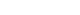precisely when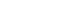Addition and multiplication of integers can be defined in terms of the equivalent operations on the natural numbers; denoting by [(a,b)] the equivalence class having (a,b) as a member, one has: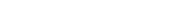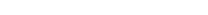The negation (or additive inverse) of an integer is obtained by reversing the order of the pair:Hence subtraction can be defined as the addition of the additive inverse: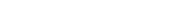The standard ordering on the integers is given by: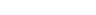iff
If and only if
In logic and related fields such as mathematics and philosophy, if and only if is a biconditional logical connective between statements....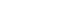It is easily verified that these definitions are independent of the choice of representatives of the equivalence classes.

Every equivalence class has a unique member that is of the form (n,0) or (0,n) (or both at once). The natural number n is identified with the class [(n,0)] (in other words the natural numbers are embedded
Embedding
In mathematics, an embedding is one instance of some mathematical structure contained within another instance, such as a group that is a subgroup....

into the integers by map sending n to [(n,0)]), and the class [(0,n)] is denoted −n (this covers all remaining classes, and gives the class [(0,0)] a second time since −0 = 0.

Thus, [(a,b)] is denoted by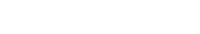If the natural numbers are identified with the corresponding integers (using the embedding mentioned above), this convention creates no ambiguity.

This notation recovers the familiar representation
Group representation
In the mathematical field of representation theory, group representations describe abstract groups in terms of linear transformations of vector spaces; in particular, they can be used to represent group elements as matrices so that the group operation can be represented by matrix multiplication...

of the integers as {... −3,−2,−1, 0, 1, 2, 3, ...}.

Some examples are:## Integers in computing

An integer is often a primitive datatype in computer languages. However, integer datatypes can only represent a subset
Subset
In mathematics, especially in set theory, a set A is a subset of a set B if A is "contained" inside B. A and B may coincide. The relationship of one set being a subset of another is called inclusion or sometimes containment...

of all integers, since practical computers are of finite capacity. Also, in the common two's complement
Two's complement
The two's complement of a binary number is defined as the value obtained by subtracting the number from a large power of two...

representation, the inherent definition of sign
Sign (mathematics)
In mathematics, the word sign refers to the property of being positive or negative. Every nonzero real number is either positive or negative, and therefore has a sign. Zero itself is signless, although in some contexts it makes sense to consider a signed zero...

distinguishes between "negative" and "non-negative" rather than "negative, positive, and 0". (It is, however, certainly possible for a computer to determine whether an integer value is truly positive.) Fixed length integer approximation datatypes (or subsets) are denoted int or Integer in several programming languages (such as Algol68, C, Java
Java (programming language)
Java is a programming language originally developed by James Gosling at Sun Microsystems and released in 1995 as a core component of Sun Microsystems' Java platform. The language derives much of its syntax from C and C++ but has a simpler object model and fewer low-level facilities...

, Delphi
Object Pascal
Object Pascal refers to a branch of object-oriented derivatives of Pascal, mostly known as the primary programming language of Embarcadero Delphi.-Early history at Apple:...

, etc.).

Variable-length representations of integers, such as bignums, can store any integer that fits in the computer's memory. Other integer datatypes are implemented with a fixed size, usually a number of bits which is a power of 2 (4, 8, 16, etc.) or a memorable number of decimal digits (e.g., 9 or 10).

## Cardinality

The cardinality of the set of integers is equal to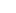(aleph-null
Aleph number
In set theory, a discipline within mathematics, the aleph numbers are a sequence of numbers used to represent the cardinality of infinite sets. They are named after the symbol used to denote them, the Hebrew letter aleph...

). This is readily demonstrated by the construction of a bijection
Bijection
A bijection is a function giving an exact pairing of the elements of two sets. A bijection from the set X to the set Y has an inverse function from Y to X. If X and Y are finite sets, then the existence of a bijection means they have the same number of elements...

, that is, a function that is injective and surjective from Z to N.

If N = {0, 1, 2, ...} then consider the function: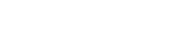{ ... (-4,8) (-3,6) (-2,4) (-1,2) (0,0) (1,1) (2,3) (3,5) ... }

If N = {1,2,3,...} then consider the function: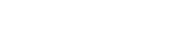{ ... (-4,8) (-3,6) (-2,4) (-1,2) (0,1) (1,3) (2,5) (3,7) ... }

If the domain is restricted to Z then each and every member of Z has one and only one corresponding member of N and by the definition of cardinal equality the two sets have equal cardinality.

## See also

• 0.999...
0.999...
In mathematics, the repeating decimal 0.999... denotes a real number that can be shown to be the number one. In other words, the symbols 0.999... and 1 represent the same number...

• Algebraic integer
Algebraic integer
In number theory, an algebraic integer is a complex number that is a root of some monic polynomial with coefficients in . The set of all algebraic integers is closed under addition and multiplication and therefore is a subring of complex numbers denoted by A...

• Canonical representation of a positive integer
• Hyperinteger
Hyperinteger
In non-standard analysis, a hyperinteger N is a hyperreal number equal to its own integer part. A hyperinteger may be either finite or infinite. A finite hyperinteger is an ordinary integer...

• Integer (computer science)
Integer (computer science)
In computer science, an integer is a datum of integral data type, a data type which represents some finite subset of the mathematical integers. Integral data types may be of different sizes and may or may not be allowed to contain negative values....

• Integer lattice
Integer lattice
In mathematics, the n-dimensional integer lattice , denoted Zn, is the lattice in the Euclidean space Rn whose lattice points are n-tuples of integers. The two-dimensional integer lattice is also called the square lattice, or grid lattice. Zn is the simplest example of a root lattice...

• Integer part
• Integer sequence
Integer sequence
In mathematics, an integer sequence is a sequence of integers.An integer sequence may be specified explicitly by giving a formula for its nth term, or implicitly by giving a relationship between its terms...

## External links

The source of this article is wikipedia, the free encyclopedia.  The text of this article is licensed under the GFDL.